# TensorFlow 模型建立與訓練¶

• 模型的建構： tf.keras.Modeltf.keras.layers

• 模型的損失函數： tf.keras.losses

• 模型的優化器： tf.keras.optimizer

• 模型的評估： tf.keras.metrics

## 模型（Model）與層（Layer）¶

Keras 有兩個重要的概念： 模型（Model）層（Layer） 。層將各種計算流程和變數進行了封裝（例如基本的全連接層(Fully Connected Layer)，CNN 的卷積層(Convolution Layer)、池化層(Pooling Layer)…等），而模型則將各種層進行組織和連接，並封裝成一個整體，描述了如何將輸入資料通過各種層以及運算而得到輸出。在需要模型呼叫的時候，使用 y_pred = model(X) 的形式即可。Keras 在 tf.keras.layers 下內建了深度學習中大量常用的的預定義層，同時也允許我們自定義層。

Keras 模型以類別的形式呈現，我們可以通過繼承 tf.keras.Model 這個 Python 類別來定義自己的模型。在繼承類別中，我們需要重寫 __init__() （建構函數，初始化）和 call(input) （模型呼叫）兩個方法，同時也可以根據需要增加自定義的方法。

class MyModel(tf.keras.Model):
def __init__(self):
super().__init__()     # Python 2 下使用 super(MyModel, self).__init__()
# 此處添加初始化程式碼（包含 call 方法中會用到的層），例如
# layer1 = tf.keras.layers.BuiltInLayer(...)
# layer2 = MyCustomLayer(...)

def call(self, input):
# 此處添加模型呼叫的程式碼（處理輸入並返回輸出），例如
# x = layer1(input)
# output = layer2(x)
return output

# 還可以添加自定義的方法


import tensorflow as tf

X = tf.constant([[1.0, 2.0, 3.0], [4.0, 5.0, 6.0]])
y = tf.constant([[10.0], [20.0]])

class Linear(tf.keras.Model):
def __init__(self):
super().__init__()
self.dense = tf.keras.layers.Dense(
units=1,
activation=None,
kernel_initializer=tf.zeros_initializer(),
bias_initializer=tf.zeros_initializer()
)

def call(self, input):
output = self.dense(input)
return output

# 以下程式碼結構與前一節類似
model = Linear()
optimizer = tf.keras.optimizers.SGD(learning_rate=0.01)
for i in range(100):
y_pred = model(X)      # 呼叫模型 y_pred = model(X) 而不是顯式寫出 y_pred = a * X + b
loss = tf.reduce_mean(tf.square(y_pred - y))
print(model.variables)


Keras 的全連接層：線性變換 + 激活函數

• units ：輸出張量的維度；

• activation ：激活函數，對應於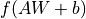中的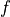，預設為無激活函數（ a(x) = x ）。常用的激活函數包括 tf.nn.relutf.nn.tanhtf.nn.sigmoid

• use_bias ：是否加入偏移量 bias ，即中的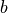。預設為 True

• kernel_initializerbias_initializer ：權重矩陣 kernel 和偏移量 bias 兩個變數的初始化器。預設為 tf.glorot_uniform_initializer 1 。設置為 tf.zeros_initializer 表示將兩個變量均初始化為全 0；

1

Keras 中的很多層都預設使用 tf.glorot_uniform_initializer 初始化變數，關於該初始化器可參考 https://www.tensorflow.org/api_docs/python/tf/keras/initializers/GlorotUniform

2

## 基礎範例：多層感知器（MLP）¶

• 使用 tf.keras.datasets 獲得資料集並預處理

• 使用 tf.keras.Modeltf.keras.layers 建構模型

• 建構模型訓練流程，使用 tf.keras.losses 計算損失函數，並使用 tf.keras.optimizer 優化模型

• 構建模型評估流程，使用 tf.keras.metrics 計算評量指標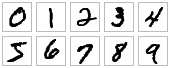MNIST 手寫體數字圖片範例

### 資料獲取及預處理： tf.keras.datasets¶

class MNISTLoader():
def __init__(self):
mnist = tf.keras.datasets.mnist
(self.train_data, self.train_label), (self.test_data, self.test_label) = mnist.load_data()
# MNIST中的圖片預設為uint8（0-255的數字）。以下程式碼將其正規化到0-1之間的浮點數，並在最後增加一維作為顏色通道
self.train_data = np.expand_dims(self.train_data.astype(np.float32) / 255.0, axis=-1)      # [60000, 28, 28, 1]
self.test_data = np.expand_dims(self.test_data.astype(np.float32) / 255.0, axis=-1)        # [10000, 28, 28, 1]
self.train_label = self.train_label.astype(np.int32)    # 
self.test_label = self.test_label.astype(np.int32)      # 
self.num_train_data, self.num_test_data = self.train_data.shape, self.test_data.shape

def get_batch(self, batch_size):
# 從資料集中隨機取出batch_size個元素並返回
index = np.random.randint(0, self.num_train_data, batch_size)
return self.train_data[index, :], self.train_label[index]


mnist = tf.keras.datasets.mnist 將從網路上自動下載 MNIST 資料集並加載。如果運行時出現網路連接錯誤，可以從 https://storage.googleapis.com/tensorflow/tf-keras-datasets/mnist.npzhttps://s3.amazonaws.com/img-datasets/mnist.npz 下載 MNIST 資料集資料。這裡使用了 mnist.npz 文件，並放置於使用者目錄的 .keras/dataset 目錄下（Windows 下使用者目錄為 C:\Users\使用者名 ，Linux 下使用者目錄為 /home/使用者名 ）。

TensorFlow 的圖像資料表示

### 模型的建構： tf.keras.Model 和 tf.keras.layers¶

class MLP(tf.keras.Model):
def __init__(self):
super().__init__()
self.flatten = tf.keras.layers.Flatten()    # Flatten層將除第一維（batch_size）以外的維度展平
self.dense1 = tf.keras.layers.Dense(units=100, activation=tf.nn.relu)
self.dense2 = tf.keras.layers.Dense(units=10)

def call(self, inputs):         # [batch_size, 28, 28, 1]
x = self.flatten(inputs)    # [batch_size, 784]
x = self.dense1(x)          # [batch_size, 100]
x = self.dense2(x)          # [batch_size, 10]
output = tf.nn.softmax(x)
return output


softmax 函數

• 該向量中的每個元素均在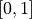之間；

• 該向量的所有元素之和為 1。

### 模型的訓練： tf.keras.losses 和 tf.keras.optimizer¶

num_epochs = 5
batch_size = 50
learning_rate = 0.001


model = MLP()


• 將這批資料送入模型，計算出模型的預測值；

• 將模型預測值與真實值進行比較，計算損失函數（loss）。這里使用 tf.keras.losses 中的交叉熵函數作為損失函數；

• 計算損失函數關於模型變數的導數；

• 將求出的導數值傳入優化器，使用優化器的 apply_gradients 方法更新模型參數以最小化損失函數（優化器的詳細使用方法見 前章 ）。

    num_batches = int(data_loader.num_train_data // batch_size * num_epochs)
for batch_index in range(num_batches):
y_pred = model(X)
loss = tf.keras.losses.sparse_categorical_crossentropy(y_true=y, y_pred=y_pred)
loss = tf.reduce_mean(loss)
print("batch %d: loss %f" % (batch_index, loss.numpy()))


tf.keras 中，有兩個交叉熵相關的損失函數 tf.keras.losses.categorical_crossentropytf.keras.losses.sparse_categorical_crossentropy 。其中 sparse 的含義是，真實的標籤值 y_true 可以直接傳入 int 類型的標籤類別。具體而言：

loss = tf.keras.losses.sparse_categorical_crossentropy(y_true=y, y_pred=y_pred)


loss = tf.keras.losses.categorical_crossentropy(
y_true=tf.one_hot(y, depth=tf.shape(y_pred)[-1]),
y_pred=y_pred
)


### 模型的評估： tf.keras.metrics¶

    sparse_categorical_accuracy = tf.keras.metrics.SparseCategoricalAccuracy()
for batch_index in range(num_batches):
start_index, end_index = batch_index * batch_size, (batch_index + 1) * batch_size
print("test accuracy: %f" % sparse_categorical_accuracy.result())


test accuracy: 0.947900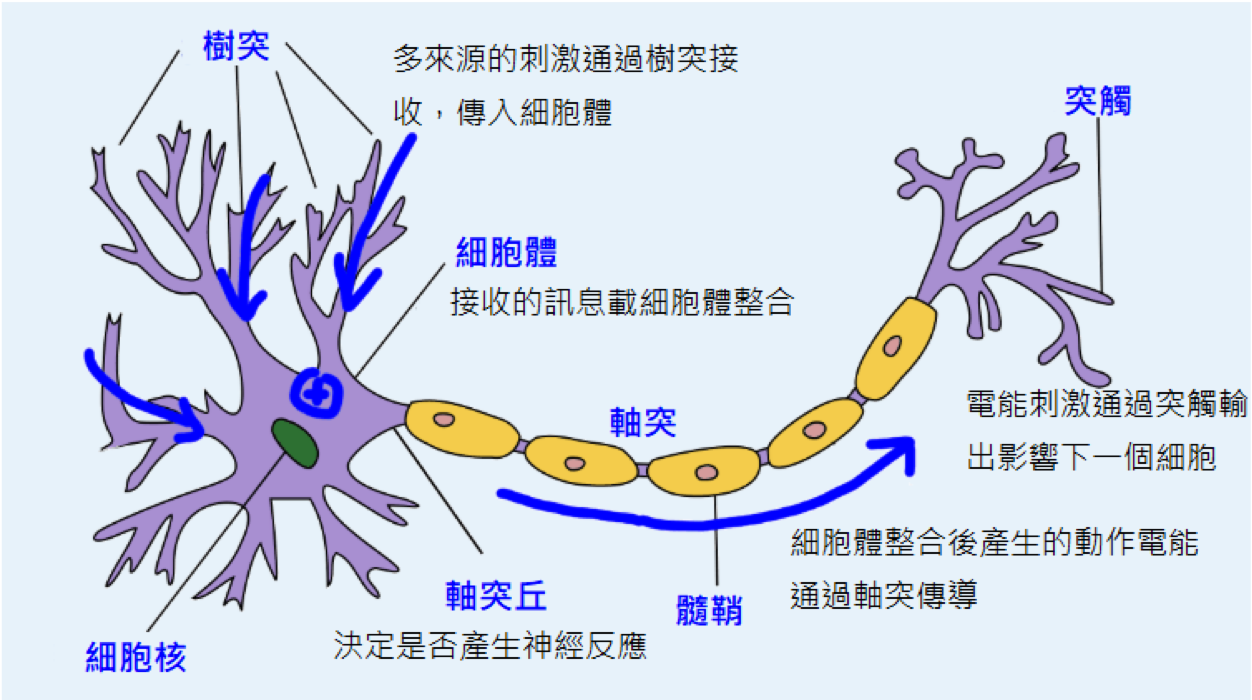3

## 卷積神經網路（CNN）¶

### 使用Keras實現卷積神經網路¶

class CNN(tf.keras.Model):
def __init__(self):
super().__init__()
self.conv1 = tf.keras.layers.Conv2D(
filters=32,             # 卷積層神經元（卷積核）數目
kernel_size=[5, 5],     # 接受區的大小
activation=tf.nn.relu   # 激活函数
)
self.pool1 = tf.keras.layers.MaxPool2D(pool_size=[2, 2], strides=2)
self.conv2 = tf.keras.layers.Conv2D(
filters=64,
kernel_size=[5, 5],
activation=tf.nn.relu
)
self.pool2 = tf.keras.layers.MaxPool2D(pool_size=[2, 2], strides=2)
self.flatten = tf.keras.layers.Reshape(target_shape=(7 * 7 * 64,))
self.dense1 = tf.keras.layers.Dense(units=1024, activation=tf.nn.relu)
self.dense2 = tf.keras.layers.Dense(units=10)

def call(self, inputs):
x = self.conv1(inputs)                  # [batch_size, 28, 28, 32]
x = self.pool1(x)                       # [batch_size, 14, 14, 32]
x = self.conv2(x)                       # [batch_size, 14, 14, 64]
x = self.pool2(x)                       # [batch_size, 7, 7, 64]
x = self.flatten(x)                     # [batch_size, 7 * 7 * 64]
x = self.dense1(x)                      # [batch_size, 1024]
x = self.dense2(x)                      # [batch_size, 10]
output = tf.nn.softmax(x)
return output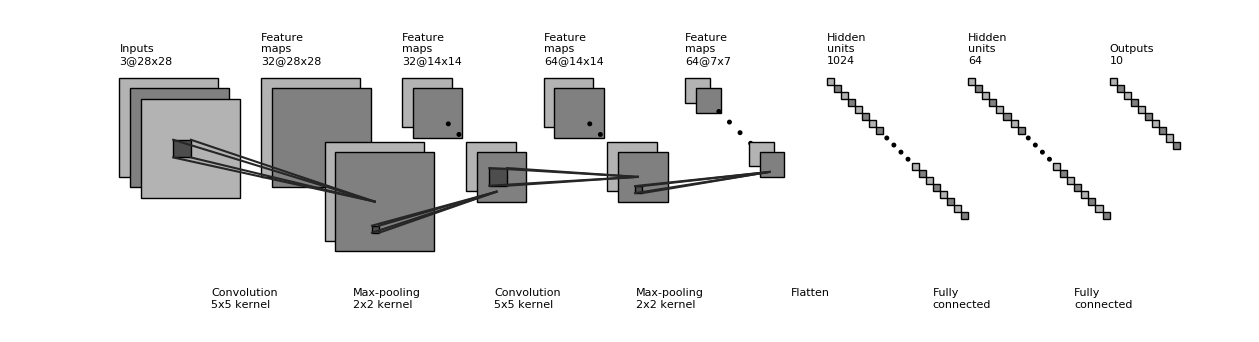test accuracy: 0.988100


### 使用Keras中預定義的典型卷積神經網路結構¶

tf.keras.applications 中有一些預定義好的典型卷積神經網路結構，如 VGG16VGG19ResNetMobileNet 等。我們可以直接呼叫這些典型的卷積神經網路結構（甚至載入預訓練的參數），而無需手動定義網路結構。

model = tf.keras.applications.MobileNetV2()


• input_shape ：輸入張量的形狀（不含第一維的Batch），大多預設為 224 × 224 × 3 。一般而言，模型對輸入張量的大小有下限，長和寬至少為 32 × 3275 × 75

• include_top ：在網路的最後是否包含全連接層，默認為 True

• weights ：預訓練權重值，預設為 'imagenet' ，即為當前模型載入在ImageNet資料集上預訓練的權重值。如需隨機初始化變數可設為 None

• classes ：分類數，預設為1000。修改該參數需要 include_top 參數為 Trueweights 參數為 None

對於一些預定義的經典模型，其中的某些層（例如BatchNormalization ）在訓練和測試時的行為是不同的（可以參考這篇文章<https://zhuanlan.zhihu.com/p/64310188>_ ）。因此，在訓練模型時，需要手動設置訓練狀態，告訴模型“我現在是處於訓練模型的階段”。可以通過

.. code-block:: python

tf.keras.backend.set_learning_phase(True)

進行設置，也可以在調用模型時通過將 training 參數設為 True 來設置。

import tensorflow as tf
import tensorflow_datasets as tfds

num_epoch = 5
batch_size = 50
learning_rate = 0.001

dataset = dataset.map(lambda img, label: (tf.image.resize(img, (224, 224)) / 255.0, label)).shuffle(1024).batch(batch_size)
model = tf.keras.applications.MobileNetV2(weights=None, classes=5)
for e in range(num_epoch):
for images, labels in dataset:
labels_pred = model(images, training=True)
loss = tf.keras.losses.sparse_categorical_crossentropy(y_true=labels, y_pred=labels_pred)
loss = tf.reduce_mean(loss)
print("loss %f" % loss.numpy())
print(labels_pred)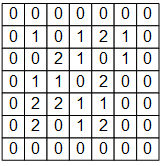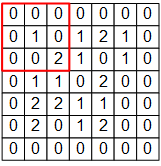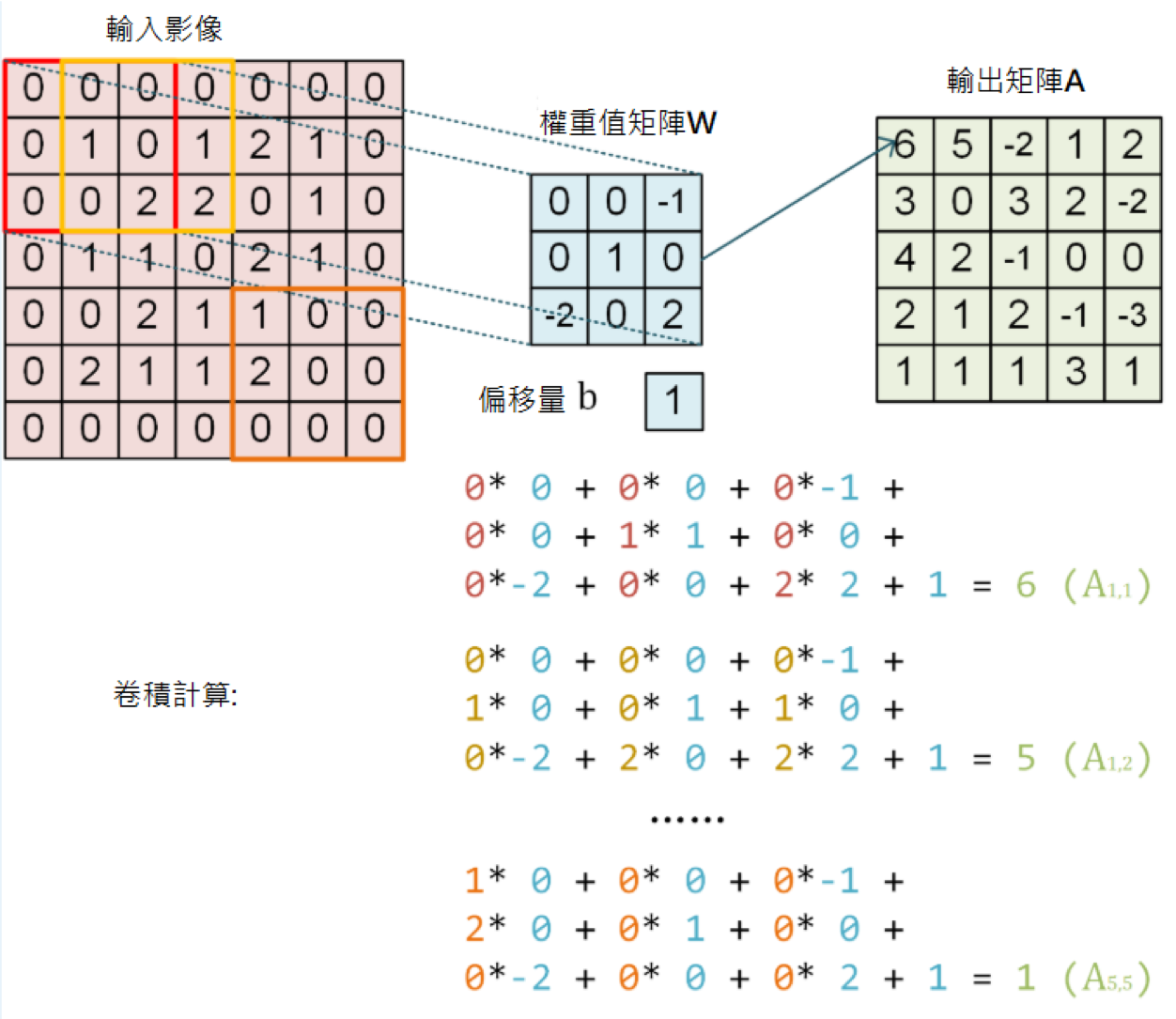# TensorFlow 的圖片表示為 [圖片數目，長，寬，色彩通道數] 的四維張量
# 這裡我們的輸入圖片 image 的張量形狀為 [1, 7, 7, 1]
image = np.array([[
[0, 0, 0, 0, 0, 0, 0],
[0, 1, 0, 1, 2, 1, 0],
[0, 0, 2, 2, 0, 1, 0],
[0, 1, 1, 0, 2, 1, 0],
[0, 0, 2, 1, 1, 0, 0],
[0, 2, 1, 1, 2, 0, 0],
[0, 0, 0, 0, 0, 0, 0]
]], dtype=np.float32)
image = np.expand_dims(image, axis=-1)
W = np.array([[
[ 0, 0, -1],
[ 0, 1, 0 ],
[-2, 0, 2 ]
]], dtype=np.float32)
b = np.array(, dtype=np.float32)


model = tf.keras.models.Sequential([
tf.keras.layers.Conv2D(
filters=1,              # 卷積層神經元（卷積核）數目
kernel_size=[3, 3],     # 接受區大小
kernel_initializer=tf.constant_initializer(W),
bias_initializer=tf.constant_initializer(b)
)]
)


output = model(image)
print(tf.squeeze(output))


tf.Tensor(
[[ 6.  5. -2.  1.  2.]
[ 3.  0.  3.  2. -2.]
[ 4.  2. -1.  0.  0.]
[ 2.  1.  2. -1. -3.]
[ 1.  1.  1.  3.  1.]], shape=(5, 5), dtype=float32)


4

## 循環神經網路（RNN）¶

I am a studen


class DataLoader():
def __init__(self):
path = tf.keras.utils.get_file('nietzsche.txt',
origin='https://s3.amazonaws.com/text-datasets/nietzsche.txt')
with open(path, encoding='utf-8') as f:
self.chars = sorted(list(set(self.raw_text)))
self.char_indices = dict((c, i) for i, c in enumerate(self.chars))
self.indices_char = dict((i, c) for i, c in enumerate(self.chars))
self.text = [self.char_indices[c] for c in self.raw_text]

def get_batch(self, seq_length, batch_size):
seq = []
next_char = []
for i in range(batch_size):
index = np.random.randint(0, len(self.text) - seq_length)
seq.append(self.text[index:index+seq_length])
next_char.append(self.text[index+seq_length])
return np.array(seq), np.array(next_char)       # [batch_size, seq_length], [num_batch]output, state = self.cell(inputs[:, t, :], state) 圖示

class RNN(tf.keras.Model):
def __init__(self, num_chars, batch_size, seq_length):
super().__init__()
self.num_chars = num_chars
self.seq_length = seq_length
self.batch_size = batch_size
self.cell = tf.keras.layers.LSTMCell(units=256)
self.dense = tf.keras.layers.Dense(units=self.num_chars)

def call(self, inputs, from_logits=False):
inputs = tf.one_hot(inputs, depth=self.num_chars)       # [batch_size, seq_length, num_chars]
state = self.cell.get_initial_state(batch_size=self.batch_size, dtype=tf.float32)
for t in range(self.seq_length):
output, state = self.cell(inputs[:, t, :], state)
logits = self.dense(output)
if from_logits:
return logits
else:
return tf.nn.softmax(logits)


    num_batches = 1000
seq_length = 40
batch_size = 50
learning_rate = 1e-3


• DataLoader 中隨機取一批訓練資料；

• 將這批資料送入模型，計算出模型的預測值；

• 將模型預測值與真實值進行比較，計算損失函數（loss）；

• 計算損失函數關於模型變數的導數；

• 使用優化器更新模型參數以最小化損失函數。

    data_loader = DataLoader()
for batch_index in range(num_batches):
y_pred = model(X)
loss = tf.keras.losses.sparse_categorical_crossentropy(y_true=y, y_pred=y_pred)
loss = tf.reduce_mean(loss)
print("batch %d: loss %f" % (batch_index, loss.numpy()))


    def predict(self, inputs, temperature=1.):
batch_size, _ = tf.shape(inputs)
logits = self(inputs, from_logits=True)
prob = tf.nn.softmax(logits / temperature).numpy()
return np.array([np.random.choice(self.num_chars, p=prob[i, :])
for i in range(batch_size.numpy())])


    X_, _ = data_loader.get_batch(seq_length, 1)
for diversity in [0.2, 0.5, 1.0, 1.2]:
X = X_
print("diversity %f:" % diversity)
for t in range(400):
y_pred = model.predict(X, diversity)
X = np.concatenate([X[:, 1:], np.expand_dims(y_pred, axis=1)], axis=-1)
print("\n")


diversity 0.200000:
conserted and conseive to the conterned to it is a self--and seast and the selfes as a seast the expecience and and and the self--and the sered is a the enderself and the sersed and as a the concertion of the series of the self in the self--and the serse and and the seried enes and seast and the sense and the eadure to the self and the present and as a to the self--and the seligious and the enders

diversity 0.500000:
can is reast to as a seligut and the complesed
has fool which the self as it is a the beasing and us immery and seese for entoured underself of the seless and the sired a mears and everyther to out every sone thes and reapres and seralise as a streed liees of the serse to pease the cersess of the selung the elie one of the were as we and man one were perser has persines and conceity of all self-el

diversity 1.000000:
entoles by
their lisevers de weltaale, arh pesylmered, and so jejurted count have foursies as is
descinty iamo; to semplization refold, we dancey or theicks-welf--atolitious on his
such which
here
oth idey of pire master, ie gerw their endwit in ids, is an trees constenved mase commars is leed mad decemshime to the mor the elige. the fedies (byun their ope wopperfitious--antile and the it as the f

diversity 1.200000:
inselfy!--ie the rads incults of to prusely le]enfes patuateded:.--a coud--theiritibaior "nrallysengleswout peessparify oonsgoscess teemind thenry ansken suprerial mus, cigitioum: 4reas. whouph: who
eved
arn inneves to sya" natorne. hag open reals whicame oderedte,[fingo is
zisternethta simalfule dereeg hesls lang-lyes thas quiin turjentimy; periaspedey tomm--whach

5

• 對輸入向量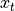通過矩陣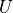進行線性變換，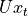與狀態 s 具有相同的維度；

•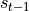通過矩陣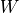進行線性變換，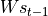與狀態 s 具有相同的維度；

• 將上述得到的兩個向量相加並通過激活函數，作為當前狀態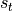的值，即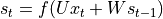。也就是說，當前狀態的值是上一個狀態的值和當前輸入進行某種資訊整合而產生的；

• 對當前狀態通過矩陣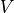進行線性變換，得到當前時間的輸出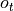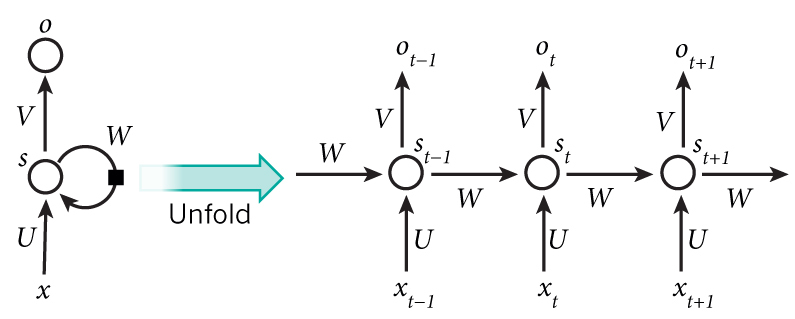## 深度強化學習（DRL）¶

import gym

env = gym.make('CartPole-v1')       # 實例化一個遊戲環境，參數為遊戲名稱
state = env.reset()                 # 初始化環境，獲得初始狀態
while True:
env.render()                    # 對當前影像進行渲染，繪圖到螢幕
action = model.predict(state)   # 假設我們有一個訓練好的模型，能夠通過當前狀態預測出這時應該進行的動作
next_state, reward, done, info = env.step(action)   # 讓環境執行動作，獲得執行完動作的下一個狀態，動作的獎勵，遊戲是否已結束以及額外資訊
if done:                        # 如果遊戲結束則退出循環
break


import tensorflow as tf
import numpy as np
import gym
import random
from collections import deque

num_episodes = 500              # 遊戲訓練的總episode數量
num_exploration_episodes = 100  # 探索過程所占的episode數量
max_len_episode = 1000          # 每個episode的最大回合數
batch_size = 32                 # 批次大小
learning_rate = 1e-3            # 學習率
gamma = 1.                      # 折扣因子
initial_epsilon = 1.            # 探索起始時的探索率
final_epsilon = 0.01            # 探索終止時的探索率


class QNetwork(tf.keras.Model):
def __init__(self):
super().__init__()
self.dense1 = tf.keras.layers.Dense(units=24, activation=tf.nn.relu)
self.dense2 = tf.keras.layers.Dense(units=24, activation=tf.nn.relu)
self.dense3 = tf.keras.layers.Dense(units=2)

def call(self, inputs):
x = self.dense1(inputs)
x = self.dense2(x)
x = self.dense3(x)
return x

def predict(self, inputs):
q_values = self(inputs)
return tf.argmax(q_values, axis=-1)


if __name__ == '__main__':
env = gym.make('CartPole-v1')       # 實例化一個遊戲環境，參數為遊戲名稱
model = QNetwork()
replay_buffer = deque(maxlen=10000) # 使用一個 deque 作為 Q Learning 的經驗回放池
epsilon = initial_epsilon
for episode_id in range(num_episodes):
state = env.reset()             # 初始化環境，獲得初始狀態
epsilon = max(                  # 計算當前探索率
initial_epsilon * (num_exploration_episodes - episode_id) / num_exploration_episodes,
final_epsilon)
for t in range(max_len_episode):
env.render()                                # 對當前影像進行渲染，繪圖到影像
if random.random() < epsilon:               # epsilon-greedy 探索策略，以 epsilon 的機率選擇隨機動作
action = env.action_space.sample()      # 選擇隨機動作（探索）
else:
action = model.predict(np.expand_dims(state, axis=0)).numpy()   # 選擇模型計算出的 Q Value 最大的動作
action = action

# 讓環境執行動作，獲得執行完動作的下一個狀態，動作的獎勵，遊戲是否已結束以及額外資訊
next_state, reward, done, info = env.step(action)
# 如果遊戲Game Over，給予大的負獎勵
reward = -10. if done else reward
# 將(state, action, reward, next_state)的四元組（外加 done 標籤表示是否結束）放入經驗回放池
replay_buffer.append((state, action, reward, next_state, 1 if done else 0))
# 更新當前 state
state = next_state

if done:                                    # 遊戲結束則退出本輪循環，進行下一個 episode
print("episode %d, epsilon %f, score %d" % (episode_id, epsilon, t))
break

if len(replay_buffer) >= batch_size:
# 從經驗回放池中隨機取一個批次的四元組，並分別轉換為 NumPy 陣列
batch_state, batch_action, batch_reward, batch_next_state, batch_done = zip(
*random.sample(replay_buffer, batch_size))
batch_state, batch_reward, batch_next_state, batch_done = \
[np.array(a, dtype=np.float32) for a in [batch_state, batch_reward, batch_next_state, batch_done]]
batch_action = np.array(batch_action, dtype=np.int32)

q_value = model(batch_next_state)
y = batch_reward + (gamma * tf.reduce_max(q_value, axis=1)) * (1 - batch_done)  # 計算 y 值
loss = tf.keras.losses.mean_squared_error(  # 最小化 y 和 Q-value 的距離
y_true=y,
y_pred=tf.reduce_sum(model(batch_state) * tf.one_hot(batch_action, depth=2), axis=1)
)


• 磚塊的顏色資訊並不是很重要，畫面轉換成灰階也不影響操作，因此可以去除狀態中的顏色資訊（即將圖片轉為灰階表示）；

• 小球移動的資訊很重要，如果只知道單一張影像畫面而不知道小球往哪邊移動，即使是人也很難判斷擋板應當移動的方向。因此，必須在狀態中加入小球運動方向的資訊。一個簡單的方式是將當前影像與前面幾張影像畫面進行疊加，得到一個 210 * 160 * X （X 為疊加影格數）的狀態表示；

• 每個影格數的分辨率不需要特別高，只要能大致瞭解方塊、小球和擋板的位置以做出決策即可，因此對於每張影像的長寬可做適當壓縮。

## Keras Pipeline *¶

### Keras Sequential/Functional API 模式建立模型¶

        model = tf.keras.models.Sequential([
tf.keras.layers.Flatten(),
tf.keras.layers.Dense(100, activation=tf.nn.relu),
tf.keras.layers.Dense(10),
tf.keras.layers.Softmax()
])


        inputs = tf.keras.Input(shape=(28, 28, 1))
x = tf.keras.layers.Flatten()(inputs)
x = tf.keras.layers.Dense(units=100, activation=tf.nn.relu)(x)
x = tf.keras.layers.Dense(units=10)(x)
outputs = tf.keras.layers.Softmax()(x)
model = tf.keras.Model(inputs=inputs, outputs=outputs)


### 使用 Keras Model 的 compile 、 fit 和 evaluate 方法訓練和評估模型¶

    model.compile(
loss=tf.keras.losses.sparse_categorical_crossentropy,
metrics=[tf.keras.metrics.sparse_categorical_accuracy]
)


tf.keras.Model.compile 接受 3 個重要的參數：

• oplimizer ：優化器，可從 tf.keras.optimizers 中選擇；

• loss ：損失函數，可從 tf.keras.losses 中選擇；

• metrics ：評量指標，可從 tf.keras.metrics 中選擇。

    model.fit(data_loader.train_data, data_loader.train_label, epochs=num_epochs, batch_size=batch_size)


tf.keras.Model.fit 接受 5 個重要的參數：

• x ：訓練資料；

• y ：目標資料（資料標籤）；

• epochs ：將訓練資料疊代多少遍；

• batch_size ：批次的大小；

• validation_data ：驗證資料，可用於在訓練過程中監控模型的性能。

Keras 支援使用 tf.data.Dataset 進行訓練，詳見 tf.data

    print(model.evaluate(data_loader.test_data, data_loader.test_label))


## 自定義層、損失函數和評量指標 *¶

### 自定義層¶

class MyLayer(tf.keras.layers.Layer):
def __init__(self):
super().__init__()
# 初始化程式碼

def build(self, input_shape):     # input_shape 是一個 TensorShape 類型對象，提供輸入的形狀
# 在第一次使用該層的時候呼叫該部分程式碼，在這裡創建變數可以使得變數的形狀自適應輸入
# 而不需要使用者額外指定變數形狀。
# 如果已經可以完全確定變數的形狀，也可以在__init__部分創建變數

def call(self, inputs):
# 模型呼叫的程式碼（處理輸入並返回輸出）
return output


class LinearLayer(tf.keras.layers.Layer):
def __init__(self, units):
super().__init__()
self.units = units

def build(self, input_shape):     # 這裡 input_shape 是第一次運行call()時參數inputs的形狀
shape=[input_shape[-1], self.units], initializer=tf.zeros_initializer())
shape=[self.units], initializer=tf.zeros_initializer())

def call(self, inputs):
y_pred = tf.matmul(inputs, self.w) + self.b
return y_pred


class LinearModel(tf.keras.Model):
def __init__(self):
super().__init__()
self.layer = LinearLayer(units=1)

def call(self, inputs):
output = self.layer(inputs)
return output


### 自定義損失函數和評量指標¶

class MeanSquaredError(tf.keras.losses.Loss):
def call(self, y_true, y_pred):
return tf.reduce_mean(tf.square(y_pred - y_true))


class SparseCategoricalAccuracy(tf.keras.metrics.Metric):
def __init__(self):
super().__init__()

def update_state(self, y_true, y_pred, sample_weight=None):
values = tf.cast(tf.equal(y_true, tf.argmax(y_pred, axis=-1, output_type=tf.int32)), tf.int32)

def result(self):
return self.count / self.total

LeCun1998
1. LeCun, L. Bottou, Y. Bengio, and P. Haffner. “Gradient-based learning applied to document recognition.” Proceedings of the IEEE, 86(11):2278-2324, November 1998. http://yann.lecun.com/exdb/mnist/

Graves2013

Graves, Alex. “Generating Sequences With Recurrent Neural Networks.” ArXiv:1308.0850 [Cs], August 4, 2013. http://arxiv.org/abs/1308.0850.

Mnih2013

Mnih, Volodymyr, Koray Kavukcuoglu, David Silver, Alex Graves, Ioannis Antonoglou, Daan Wierstra, and Martin Riedmiller. “Playing Atari with Deep Reinforcement Learning.” ArXiv:1312.5602 [Cs], December 19, 2013. http://arxiv.org/abs/1312.5602.